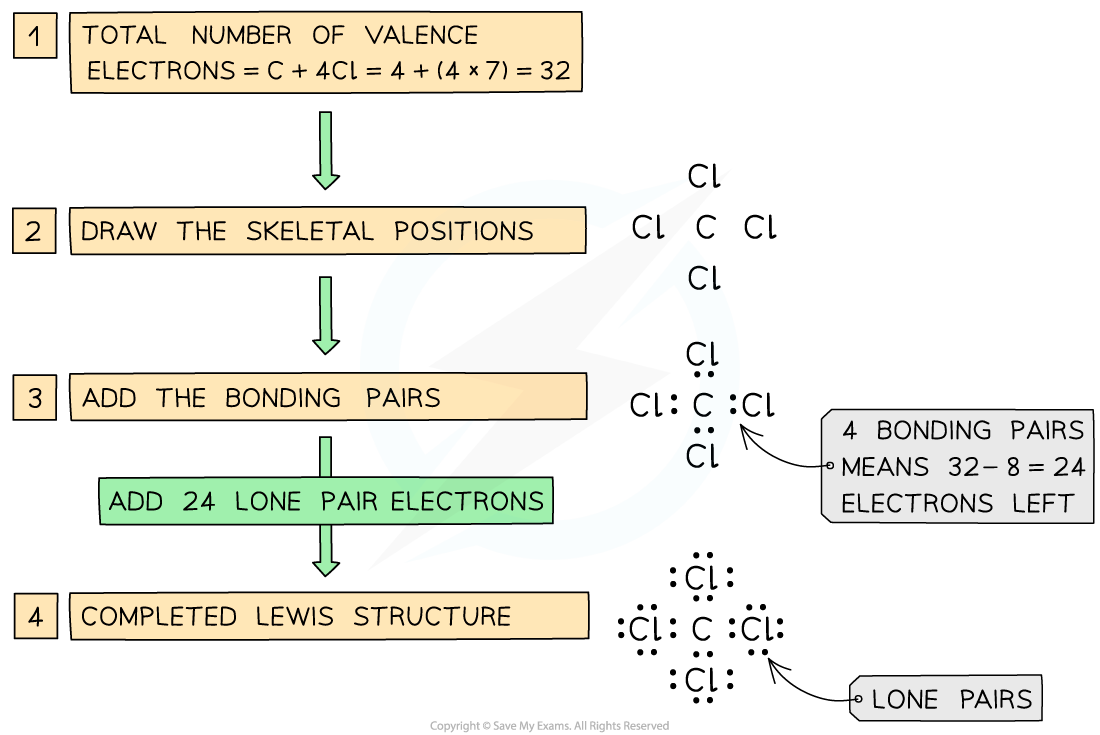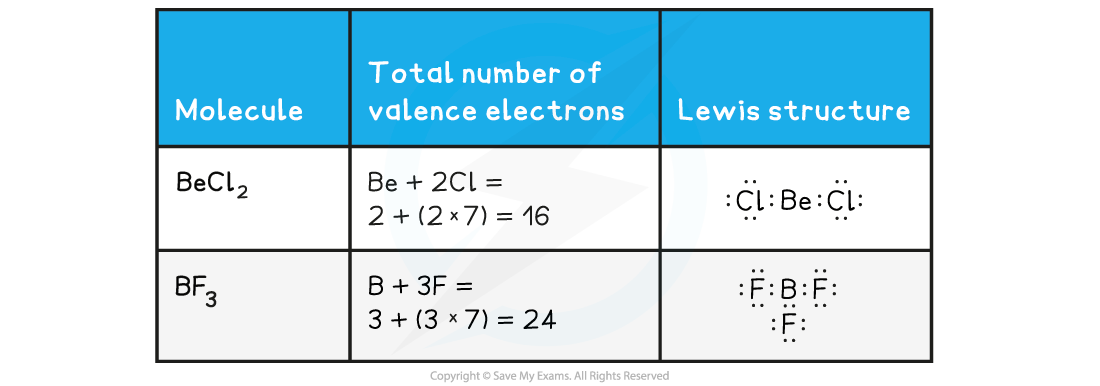# IB DP Chemistry: SL复习笔记4.1.6 Lewis Structures

### Lewis Structures

• Lewis structures are simplified electron shell diagrams and show pairs of electrons around atoms.
• A pair of electrons can be represented by dots, crosses, a combination of dots and crosses or by a line. For example, chlorine can be shown as:Different Lewis Structures for chlorine molecules

• Note: Cl–Cl is not a Lewis structure, since it does not show all the electron pairs.
• The “octet rule” refers to the tendency of atoms to gain a valence shell with a total of 8 electrons

#### Steps for drawing Lewis Structures

1. Count the total number of valence
2. Draw the skeletal structure to show how many atoms are linked to each other.
3. Use a pair of crosses or dot/cross to put an electron pair in each bond between the atoms.
4. Add more electron pairs to complete the octets around the atoms ( except H which has 2 electrons)
5. If there are not enough electrons to complete the octets, form double/triple bonds.
6. Check the total number of electrons in the finished structure is equal to the total number of valence electrons

#### Worked Example

Draw a Lewis structure for CCl4Steps in drawing the Lewis Structure for CCl4

#### Further examples of Lewis structures

• Follow the steps for drawing Lewis structures for these common molecules

### Incomplete Octets

• For elements below atomic number 20 the octet rule states that the atoms try to achieve 8 electrons in their valence shells, so they have the same electron configuration as a noble gas
• However, there are some elements that are exceptions to the octet rule, such a H, Li, Be, B and Al
• H can achieve a stable arrangement by gaining an electron to become 1s2, the same structure as the noble gas helium
• Li does the same, but losing an electron and going from 1s22sto 1s2 to become a Li+ ion
• Be from group 2, has two valence electrons and forms stable compounds with just four electrons in the valence shell
• B and Al in group 13 have 3 valence electrons and can form stable compounds with only 6 valence electrons
• There are two examples of Lewis structures with incomplete octets you should know, BeCl2 and BF3:

Incomplete Octets Examples• Test your understanding of Lewis diagrams in the following example:

#### Worked Example

How many electrons are in the 2-aminoethanoic acid molecule?A.  18

B.  20

C.  28

D.  30

The correct option is D.

• You must count the lone pairs on N and O as well as the bonding pairs. There are 5 ‘hidden’ pairs of bonding electrons in the OH, CH2 and NHgroups. Hydrogen does not follow the octet rule.